NCERT Solutions for Class 8 Maths Chapter 2 Linear Equations in One Variable Ex 2.1 are part of NCERT Solutions for Class 8 Maths. Here we have given NCERT Solutions for Class 8 Maths Chapter 2 Linear Equations in One Variable Ex 2.1.

 Board CBSE Textbook NCERT Class Class 8 Subject Maths Chapter Chapter 2 Chapter Name Linear Equations in One Variable Exercise Ex 2.1, Ex 2.2, Ex 2.3, Ex 2.4, Ex 2.5, Ex 2.6 Number of Questions Solved 1 Category NCERT Solutions

## NCERT Solutions for Class 8 Maths Chapter 2 Linear Equations in One Variable Ex 2.1

Question 1.
Solve the following equations: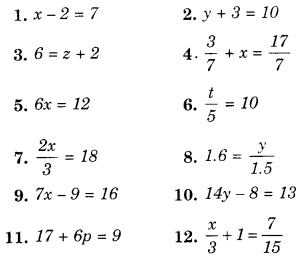Solution.
1. x-2 = 7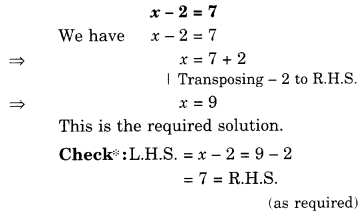2. y+3=10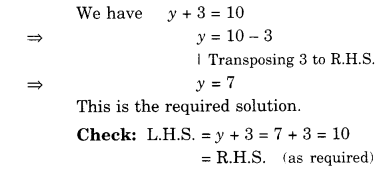3. 6=z+2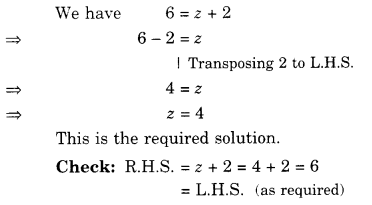4. $$\frac { 3 }{ 7 } +x=\frac { 17 }{ 7 }$$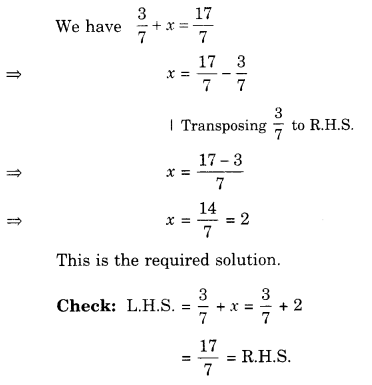5. 6x=12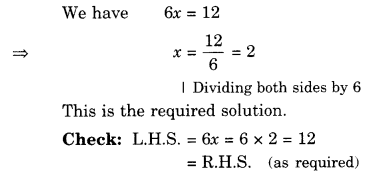6. $$\frac { t }{ 5 } =10$$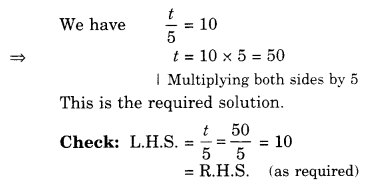7. $$\frac { 2x }{ 3 } =18$$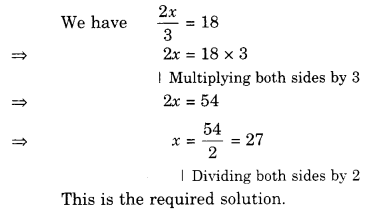8. $$1.6=\frac { y }{ 1.5 }$$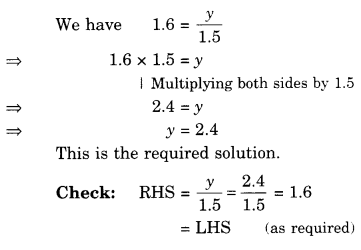9. 7x-9=1610. 14y-8=13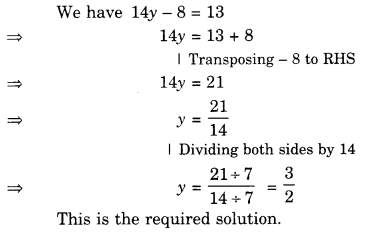11. 17+6p=912. $$\frac { x }{ 3 } +1=\frac { 7 }{ 15 }$$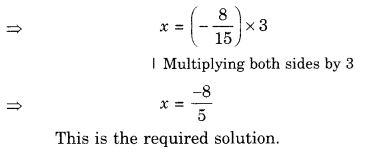We hope the NCERT Solutions for Class 8 Maths Chapter 2 Linear Equations in One Variable Ex 2.1 help you. If you have any query regarding NCERT Solutions for Class 8 Maths Chapter 2 Linear Equations in One Variable Ex 2.1, drop a comment below and we will get back to you at the earliest.